## WitCH 16: The Root of Our Problem

This WitCH comes from one of our favourites, the Complex Numbers chapter from Cambridge’s Specialist Mathematics 3 & 4 (2019). It is not as deep or as beWitCHing as other aspects of the chapter. But, it’s still an impressive WitCH.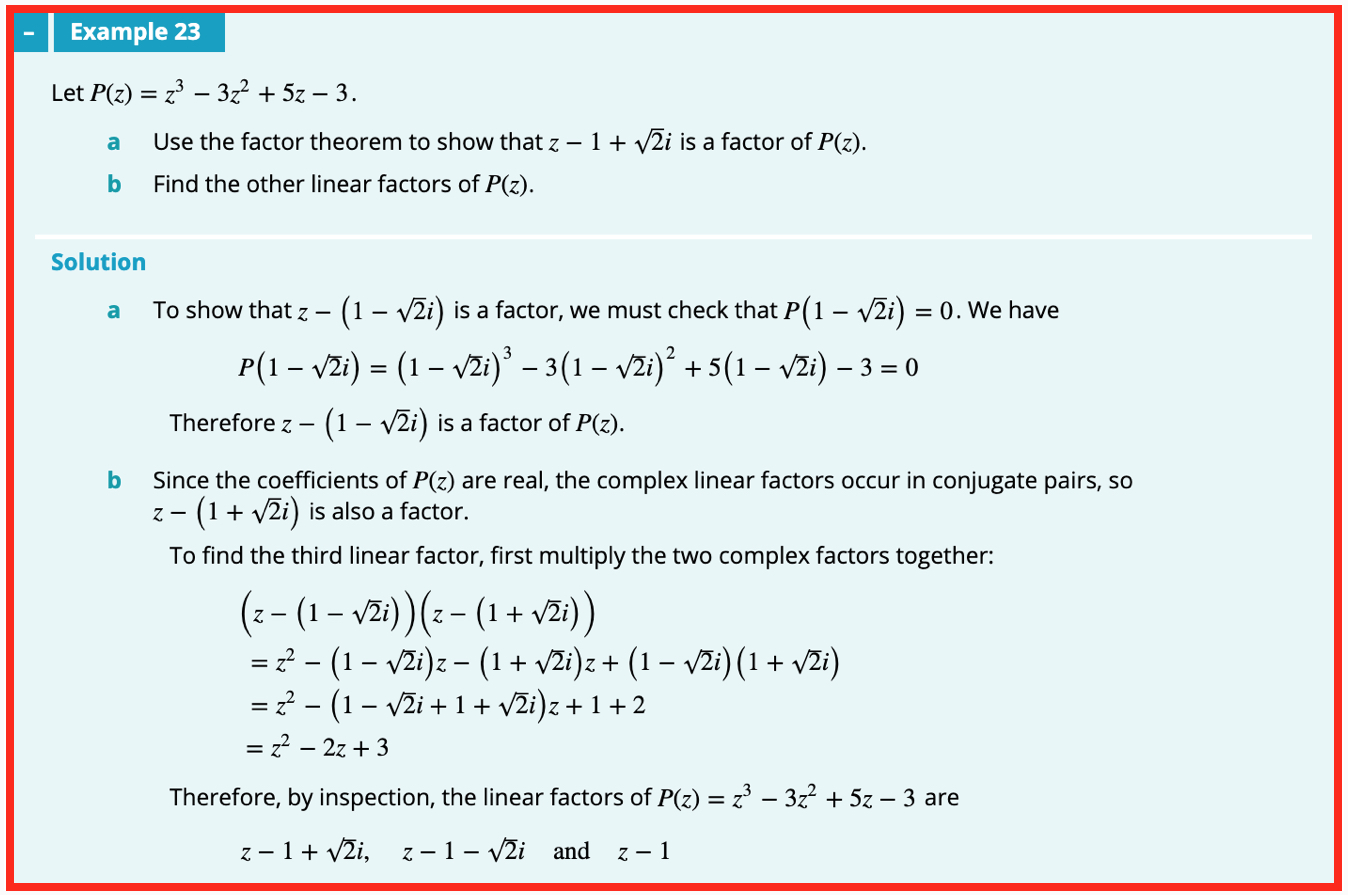## Update (11/08/19)

I guess if you’re gonna suggest a painful, ass-backwards method to solve a problem, you may as well fake the solution:

• Checking directly that P(1 – i√2) = 0 involves expanding a cubic, and more, which the text does in one single magic line.
• The painful multiplication of the products for part b is much more naturally and easily done as a difference of two squares: (z – 1 – i√2)(z – 1 + i√2) = (z – 1)2 + 2, etc.
• After all that the third factor, z – 1, is determined “by inspection”? Inspection of what?

AS RF notes, it is much easier to spot that z = 1 solves the cubic. Then some easy factoring (without long division …) gives P = (z – 1)(z2 – 2z + 3). Completing the square then leads to the linear factors, answering both parts of the question in the reverse, and natural, order.

Alternatively, as John notes, the difference of two squares calculation shows that if z – 1 + i√2 is a factor of P then so is the quadratic z^2 – 2z + 3. That this is so can then be checked (without long division …), giving P = (z – 1)(z^2 – 2z + 3), and so on, as before.

## Inferiority Complex

This one is long, a real Gish gallop. Question 4, Part 2 from the 2017 VCE Specialist Mathematics Exam 2 is a mess. The Examiners’ Report is, predictably, worse.

Part (a) of Question 4 is routine, requiring students to express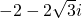in polar form. One wonders how a quarter of the students could muck up this easy 1-mark question, but the question is fine.

The issues begin with 4(b), for which students are required to

Show that the roots ofareand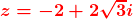.

The question can be answered with an easy application of completing the square or the quadratic formula. So, why did almost half of the students get it wrong? Were so many students really so clueless? Perhaps, but there is good reason to suspect a different source of the cluelessness.

The Examiners’ Report indicates three general issues with students’ answers. First,

students confused factors with solutions or did not proceed beyond factorising the quadratic.

Maybe the students were confused, but maybe not. Maybe some students simply thought that, once having factorised the quadratic, the microstep to then write “Therefore z = …”, to note the roots written on the exam in front of them, was too trivial in response to a 1 mark question.

Second, some students reportedly erred by

not showing key steps in their solution.

Really? The Report includes the following calculation as a sample solution:Was this whole tedious, snail-paced computation required for one measly mark? It’s impossible to tell, but the Report remarks generally on ‘show that’ questions that

all steps that led to the given result needed to be clearly and logically set out.

As we have noted previously, demanding “all steps” is both meaningless and utterly mad. For a year 12 advanced mathematics student the identification of the roots is pretty much immediate and a single written step should suffice. True, in 4(b) students are instructed to “show” stuff, but it’s hardly the students’ fault that what they were instructed to show is pretty trivial.

Third, and by far the most ridiculous,

some students did not correctly follow the ‘show that’ instruction … by [instead] solely verifying the solutions given by substitution.

Bullshit.

VCAA examiners love to worry that word “show”. In true Princess Bride fashion, however, the word does not mean what they think it means.

There is nothing in standard English usage nor in standard mathematical usage, nor in at least occasional VCE usage (see Q2(a)), that would distinguish “show” from “prove” in this context. And, for 4(b) above, substitution of the given values into the quadratic is a perfectly valid method of proving that the roots are as indicated.

It appears that VCE has a special non-English code, in which “show” has a narrower meaning, akin to “derive“. This cannot alter the fact that the VCE examiners’ use of the word is linguistic and mathematical crap. It also cannot alter the fact that students being penalised for not following this linguistic and mathematical crap is pedagogical and mathematical crap.

Of course all the nonsense of 4(b) could have been avoided simply by asking the students to find the roots. The examiners declined to do so, however, probably because this would have violated VCAA’s policy of avoiding asking any mathematical question with some depth or difficulty or further consequence. The result is a question amounting to no more than an infantile and infantilising ritual, penalising any student with the mathematical common sense to answer with the appropriate “well, duh”.

***************************

Onwards we trek to 4(c):

Express the roots of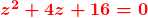in terms of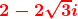.

Less than a third of students scored the mark for this question, and the Report notes that

Misunderstanding of the question was apparent in student responses. Many attempts at solutions were not expressed in terms of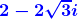as required.

Funny that. The examiners pose a question that borders on the meaningless and somehow this creates a sea of misunderstanding. Who would’ve guessed?

4(c) makes little more sense than to ask someone to write 3 in terms of 7. Given any two numbers there’s a zillion ways to “express” one number “in terms of” the other, as in 3 = 7 – 4 or whatever. Without further qualification or some accepted convention, without some agreed upon definition of “expressed in terms of”, any expression is just as valid as any other.

What was expected in 4(c)? To approach the question cleanly we can first set, as the examiners could have and should have and did not. Then, the intended answers wereand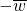.

These expressions for the roots are simple and natural, but even if one accepts a waffly interpretation of 4(c) that somehow requires “simple” solutions, there are plenty of other possible answers. The expressions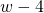andandandare all reasonable and natural, but nothing in the Examiners’ Report suggests that these or similar answers were accepted. If not, that is a very nasty cherry on top of an incredibly silly question.

***************************

The pain now temporarily lessens (though the worst is yet to come). 4(d) asks for students to show that the relationhas the cartesian form, and in 4(e) students are asked to draw this line on an Argand diagram, together with the roots of the above quadratic.

These questions are routine and ok, though 4(d) is weirdly aimless, the line obtained playing no role in the final parts of Q4. The Examiners’ Report also notes condescendingly that “the ‘show that’ instruction was generally followed”. Yes, people do tend to follow the intended road if there’s only one road.

The final part, 4(g), is also standard, requiring students to find the area of the major segment of the circle |z| = 4 cut off by the line through the roots of the quadratic. The question is straight-forward, the only real trick being to ignore the weird line from 4(d) and 4(e).

***************************

Finally, the debacle of 4(f):

The equation of the line passing through the two roots ofcan be expressed as, where. Find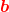in terms of.

The Report notes that

This question caused significant difficulty for students.

That’s hilarious understatement given that 99% of students scored 0/1 on the question. The further statements acknowledging and explaining and apologising for the stuff-up are unfortunately non-existent.

So, what went wrong? The answer is both obvious and depressingly familiar: the exam question is essentially meaningless. Students failed to comprehend the question because it is close to incomprehensible.

The students are asked to write b in terms of a. However, similar to 4(c) above, there are many ways to do that and how one is able to do it depends upon the initial number a chosen. The line through the two roots has equation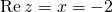. So then, for example, with a = -4 we have b = 0 and we can write b = a + 4 or b = 0 x a or whatever. If a = -5 then b = 1 and we can write b = -a – 4, and so on.

Anything of this nature is a reasonable response to the exam question as written and none of it resembles the answer in the Report. Instead, what was expected was for students to consider all complex numbers a – except those on the line itself – and to consider all associated complex b. That is, in appropriate but non-Specialist terminology, we want to determine b as a function f(a) of a, with the domain of f being most but not all of the complex plane.

With the question suitably clarified we can get down to work (none of which is indicated in the Report). Easiest is to write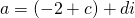. Since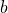must be symmetrically placed about the line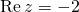, it follows that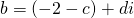. Then. This gives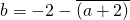, and finally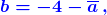which is the answer indicated in the Examiners’ Report.

In principle 4(f) is a nice question, though 1 mark is pretty chintzy for the thought required. More importantly, the exam question as written bears only the slightest resemblance to the intended question, or to anything coherent, with only the slightest, inaccurate hint of the intended generality of a and b.

99% of 2017 Specialist students have a right to be pissed off.

***************************

That’s it, we’re done. One more ridiculous VCE exam question, and one more ridiculously arrogant Report, unsullied by an ounce of self-reflection or remorse.

## WitCH 3

First, a quick note about these WitCHes. Any reasonable mathematician looking at such text extracts would immediately see the mathematical flaw(s) and would wonder how such half-baked nonsense could be published. We are aware, however, that for teachers and students, or at least Australian teachers and students, it is not nearly so easy. Since school mathematics is completely immersed in semi-sense, it is difficult to know the rules of the game. It is also perhaps difficult to know how a tentative suggestion might be received on a snarky blog such as this. We’ll just say, though we have little time for don’t-know-as-much-as-they-think textbook writers, we’re very patient with teachers and students who are honestly trying to figure out what’s what.

Now onto WitCH 3, which follows on from WitCH 2, coming from the same chapter of Cambridge’s Specialist Mathematics VCE Units 3 & 4 (2018).* The extract is below, and please post your thoughts in the comments. Also a reminder, WitCH 1 and WitCH 2 are still there, awaiting proper resolution. Enjoy.

* Cambridge is a good target, since they are the most respected of standard Australian school texts. We will, however, be whacking other publishers, and we’re always open to suggestion. Just email if you have a good WitCH candidate, or crap of any kind you wish to be attacked.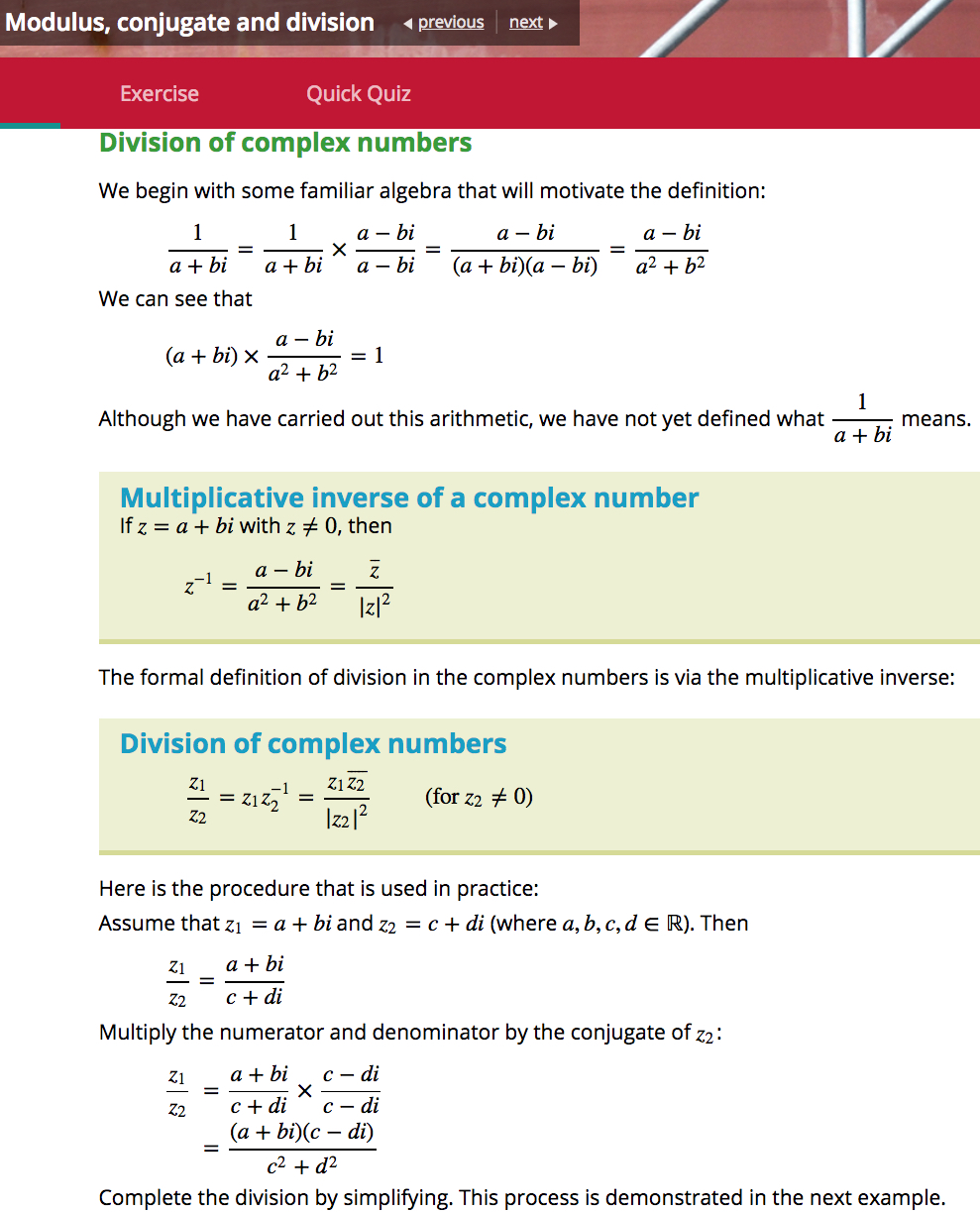## Update (06/02/19)

The above excerpt is indicative of the text’s entire chapter on complex numbers. It is such remarkably poor exposition, the foundations so understated and the direction so aimless, it is almost impossible to find one’s way back to sensible discussion.

Here is a natural framework for a Year 12 topic on complex numbers:

• First, one introduces a new numberfor which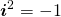.
• One then defines complex numbers, and introduces the fundamental operations of addition and multiplication.
• One then at least states, and hopefully proves, the familiar algebraic properties for complex numbers, i.e. the field laws,and so forth. All these properties are obvious or straight-forward to prove, except for the existence of multiplicative inverses; one has to prove that given any non-zero complex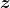there is another complex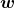with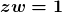.
• That is the basic complex algebra sorted, and then one can tidy up. This includes the definition of division, noting the essential role played by commutativity of multiplication.
• Then, comes the geometry of complex numbers, beginning with the definition and algebraic properties of the conjugate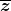and modulus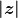,  the interpretation of these quantities in terms of the complex plane, and polar form.
• Finally, the algebra and geometry of complex numbers are related: the parallelogram interpretation of addition, the trigonometric-polar interpretation of multiplication, roots of complex numbers and so forth.

Must complex numbers be taught in this manner and in this order? No and yes. One obvious variation is to include a formal definition of a complex number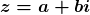as an ordered pair; as Damo remarks below, this is done as an asterisked section in Fitzpatrick and Galbraith. Though unnervingly abstract, the formal definition has the non-trivial advantage of reinforcing, almost demanding, the interpretation of complex numbers as points in the complex plane. More generally, one can emphasise more or less of the theoretical underpinnings and, to an extent, change the ordering.

But, one can only change the ordering and discard the theory so much, and no more. Complex numbers are new algebraic objects, and defining and clarifying the algebra is critical, and this fundamentally precedes the geometry.

What is the Cambridge order? The text starts off well, introducingwith,  and then immediately goes off the rails by declaring that. Then, in brief, the text includes:

(a) an invalid treatment of the square roots of negative numbers;

(b) complex addition stated, presumably defined, with the inverse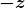introduced but not named;

(c) complex subtraction, followed by an almost invisible statement of the relevant field laws, none of which are proved or assigned as exercises;

(d) scalar multiplication;

(d) the complex plane and “the representation of the basic operations on complex numbers”;

(e) complex multiplication defined, with an almost invisible statement of field laws, none of which are proved or assigned as exercises, and with no mention of the question of multiplicative inverses;

(f) the geometry of multiplication by;

(g) the modulus of a complex number defined, with algebraic properties (including) stated and assigned as exercises;

(h) the conjugate of a complex number defined, with algebraic properties stated and either proved or assigned as exercises.

(h) Finally, as excerpted above, it’s on to reciprocals of complex numbers, multiplicative inverses in terms of modulus and conjugate, and division.

(i) This is followed by sections on polar form, de Moivre’s theorem and so forth;

(j) CAS garbage is, of course, interspersed throughout. (Which is not all Cambridge’s fault, but the text is no less ugly for that.)

At no stage in the text’s exposition is there any visible concern for emphasising or clarifying foundations, or for following a natural mathematical progression. There is too seldom an indication of what is being defined or assumed or proved.

What is the point? Yes, one can easily be overly theoretical on this topic, but this is Year 12 Specialist Mathematics. It is supposed to be special. The students have already been introduced to complex numbers in Year 11 Specialist. Indeed, much of the complex material in the Year 11 Cambridge text is repeated verbatim in the Year 12 text. Why bother? The students have already been exposed to the nuts and bolts, so why not approach the subject with some mathematical integrity, rather than just cutting and pasting aimless, half-baked nonsense?

Now, finally and briefly, some specific comments on the specific nonsense excerpted above.

• division of complex numbers has already appeared in the text, in the list of (unproved) properties of the modulus.
• the algebraic manipulation of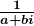is unfamiliar and unmotivated and, as is admitted way too late, is undefined. There is a place for such “let’s see” calculations – what mathematicians refer to as formal calculations –  but they have to be framed and be motivated much more carefully.
• There is no need here for a “let’s see” calculation. The critical and simple observation is thatis real. It is then a short step to realise and to prove that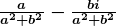acts as, and thus is, the multiplicative inverse of.
• Having finally admitted thathas not been defined, the text goes on to not define it again. The text states the multiplicative inverse of, but it is not clear whether this statement amounts to a definition or a conclusion.
• Division of complex numbers is then defined with needless subscripts and, more importantly, with no mention of the fundamental role of commutativity of multiplication.
• Throughout, the use of conjugate and modulus is muddying rather than clarifying.
• At no stage is it made clear whymakes sense in contrast to, for example, the non-sense of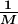for a matrix.

## WitCH 2

Well, WitCH 1 is still not satisfactorily resolved, and Tweel’s puzzle is also still out there. But, we may as well get another ball rolling.

The second in our What is this Crap Here series comes from Cambridge’s textbook Specialist Mathematics VCE Units 3 & 4 (2018). Enjoy, and please get to pondering, and posting.## Update

Thanks to Damo for their hard work below.

The main problem with the above excerpt is that it should not exist. It is pointless to introduce complex numbers with more than a sentence on complex roots, and it is almost impossible to do so in a sensible manner. The nonsense of the text’s approach is encapsulated by the equation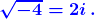This equation is best thought as false and, in the context of the excerpt above, must be thought of as meaningless. As is, thus, the discussion leading up to this equation.

How did they get there? To begin, i is introduced as a number for which i2 = -1, which is fine and good at the school level. Then, they note that the equation x2 = -1 has the two solutions i and -i, which is significantly less fine; since general complex numbers, and -i in particular, have not yet been defined, the notation -i is thus far meaningless, as is the notion of squaring this number. Still, if the sentence were more carefully worded, it would be reasonable in an introductory paragraph. The cavalier attitude to definition and meaning, however, is the sign of much worse to come.

The text continues by “declaring” that √(-1) = i, and then heads on its merry calculating way. But the calculation is complete fantasy. The declaration amounts to a (bad) definition of a specific root which cannot, in and of itself, tell us what any other root means or how it might be manipulated. So, √(-4) is as yet undefined, and the manipulation of this quantity is unjustified, as yet unjustifiable, and is best thought of as wrong.

In the real context we use √x to distinguish the positive root but it is fundamental that complex roots are multiple-valued. And, for the polynomial focus of VCE mathematics, multiple values are perfectly fine and perfectly natural. The quadratic formula remains true without change and the purportedly troublesome identityis always true (modulo the understanding that if x is a positive real then√x is now ambiguous). Moreover, with this natural interpretation, the text’s declaration that √(-1) = i is false, as is the equation √(-4) = 2i.

Admittedly, at some point it is valuable, and essential, to introduce principal values of roots, by which the text’s equation can be interpreted to be true. But principle roots are intrinsically awkward, must be introduced with great care and should only be introduced when there is a purpose. Which is not on page 1 of a school text, and arguably not ever in a school text.

Apart form the utter pointlessness and utter meaningless of the excerpt, we note:

• The text conflates the introduction of imaginary numbers in the 16th century with the introduction of the symbol i in the 18th century.
• The text implies 0 is an imaginary number, which is ok though a little peculiar.
• The real numbers and imaginary numbers are not subsets of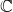.
• The characterisationsand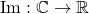are grandiose and pointless.